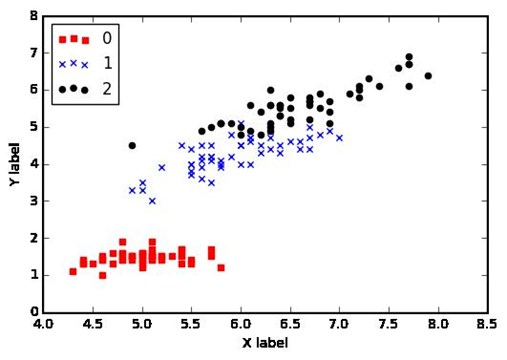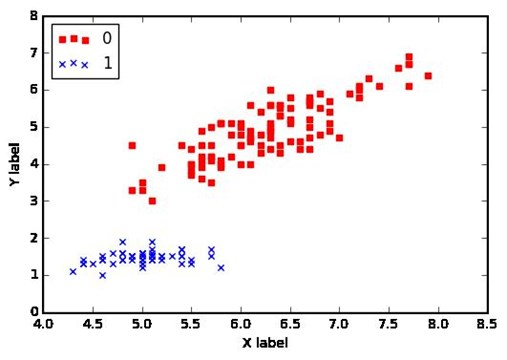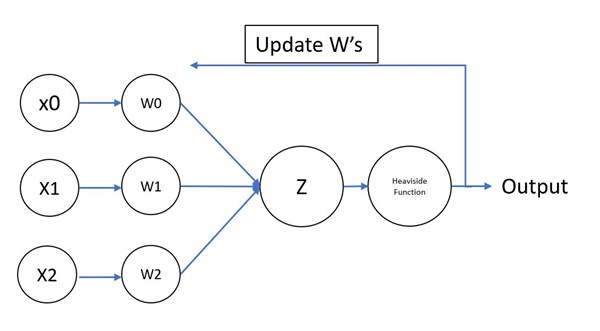## Perceptron Algorithm Part 2 Python Code | Machine Learning 101

In the previous post we discussed the theory and history behind the perceptron algorithm developed by Frank Rosenblatt. Even though this is a very basic algorithm and only capable of modeling linear relationships, it serves as a great starting point to understanding neural network machine learning models. In this post, we will implement this basic Perceptron in Python.

### Our Goal

We will be using the iris dataset made available from the sklearn library. This dataset contains 3 different types of irises and 4 features for each sample. The Y column shown below is a label either 0,1 or 2 that defines which Iris the sample is from. This will be our goal, to train a perceptron algorithm to predict the Iris (Y) given 2 features. We will be using Feature A and Feature C for our training.

Feature A Feature B Feature C Feature D Y
5.1 3.5 1.4 0.2 0
4.9 3.0 1.4 0.2 0
4.7 3.2 1.3 0.2 0
4.6 3.1 1.5 0.2 0
5.0 3.6 1.4 0.2 0

To load the data and select only the 1st and 3 column (feature A and C respectively) use the following code. Note that iris.data returns a numpy array.

``````#required library which holes the iris dataset

#our inputs will contain 2 features
X = iris.data[:, [0, 2]]
#the labels are the following
y = iris.target``````

Before we proceed let's create a scatterplot of our X and y values using the following code:

``````%matplotlib inline
import matplotlib.pyplot as plt

def plot_scatter(X,y):
colors = ["red","blue","black","yellow","green","purple","orange"]
markers = ('s', 'x', 'o', '^', 'v')

for i, yi in enumerate(np.unique(y)):
Xi = X[y==yi]
plt.scatter(Xi[:,0], Xi[:,1],
color=colors[i], marker=markers[i], label=yi)

plt.xlabel('X label')
plt.ylabel('Y label')
plt.legend(loc='upper left')

#Generate the Scatterplot
plot_scatter(X,y)``````Notice that the label 1 and 2 are not linearly separabale as there is some overlap between them both. This poses a problem for this perceptron model we are implementing. In order for our perceptron to correctly classify the labels we will aim to classify if it is a label 0 or not.

In the following code we change the labels and leave only 2 classes, label 0 and label 1,2 combined into a single class. The scatterplot now shows two classes that are linearly seperable.

``````#Classifier for y = 0
y = np.where(y == 0, 1, 0)

plot_scatter(X,y)``````### Our Model

The following image depicts the model that we will be implementing. X1 and X2 are our 2 features mentioned previously, X0 will be our bias term which will always be equal to 1 and will allow our model to shift our boundary left or right through the x axis. In short, it will improve our classifier. The sum of the multiplication of every X with its corresponding weight is Z. The heaviside function mentioned in the previous post will be used to transorm this Z into our output. In other words, the heaviside is our activation function.### The Code!

First, we need to import the libraries that will be using throughout our code. Our first import, the numpy library, is used for scientific computing and commonly used to perform vectorized operations. For example when calculating our Z value instead of performing: z = w1x1 + w2x2 + ··· wnxthe vectorized operation WT · X is much faster.

``````#import the required libraries
import numpy as np
import pandas as pd``````

### Perceptron Class

Next, we will define our Perceptron class. The constructor takes parameters that will be used in the perceptron learning rule such as the learning rate, number of iterations and the random state. The random state parameter makes our code reproductible by initializing the randomizer with the same seed.

``````class Perceptron(object):

#The constructor of our class.
def __init__(self, learningRate=0.01, n_iter=50, random_state=1):
self.learningRate = learningRate
self.n_iter = n_iter
self.random_state = random_state
self.errors_ = []``````

Next we add our Z function which computes WT · X. Our predict function takes the output of Z and uses the heaviside function to return either a 1 or a 0 label.

``````    def z(self, X):
#np.dot(X, self.w_[1:]) + self.w_
z = np.dot(X, self.weights[1:]) + self.weights
return z

def predict(self, X):
#Heaviside function. Returns 1 or 0
return np.where(self.z(X) >= 0.0, 1, 0)
``````

Almost there...

### Rosenblatt's Perceptron Training Rule Python Code

We will now implement the perceptron training rule explained in more detail in my previous post. The following fit function will take care of this. I'll explain each part of the code coming up next and tried to add as much inline comments to help you understanding the logic.

First portion is defining our fit function which takes as an input an array X and the labels y. We also initialize our radom_generator passing it our random_state parameter definied previously.

``````    def fit(self, X, y):
#for reproducing the same results
random_generator = np.random.RandomState(self.random_state)``````

Next we just extract the number of columns and rows that our input vector X contains. We are assuming the X vector does not contain a bias term. This is why we add 1 to the count of x_columns.

Step 1 of the perceptron learning rule comes next, to initialize all weights to 0 or a small random number. Here we are initializing our weights to a small random number following a normal distribution with a mean of 0 and standard deviation of 0.001.

``````        #Step 0 = Get the shape of the input vector X
#We are adding 1 to the columns for the Bias Term
x_rows, x_columns = X.shape
x_columns = x_columns+1

#Step 1 - Initialize all weights to 0 or a small random number
#weight = the weight of the Bias Term
self.weights = random_generator.normal(loc=0.0, scale=0.001, size=x_columns) ``````

Step 2 is to generate a prediction for each sample. To do this, we will loop through each row of our vector and perform a prediction for that row. Our perceptron class contains the variable n_iter which defines how many times we will loop through the input vector X.

``````        #for how many number of training iterrations where defined
for _ in range(self.n_iter):
errors = 0
for xi, y_actual in zip(X, y):
#create a prediction for the given sample xi
y_predicted = self.predict(xi)``````

Step 3 (Update the Weights) will now use our prediction to calculate how much our weights need to change.

First we calculate the delta = ∆wj = η(y(i) - ẏ(i)) xj(i)

Next, we add the delta to our weights: wj := wj + ∆wj and as we do this, each subsequent prediction shall be closer to the correct value.

For each sample in each batch we will keep count of the errors in prediction when the delta is greater than 0 and once the batch finishes, we add the error count to the errors variable.

``````                #calculte the delta
delta = self.learningRate*(y_actual - y_predicted)
#update all the weights but the bias
self.weights[1:] += delta * xi
#for the bias delta*1 = delta
self.weights += delta

#if there is an error, increase the error count for the batch
errors += int(delta != 0.0)

#add the error count of the batch to the errors variable
self.errors_.append(errors) ``````

We are all set to train our model!

### Train the Perceptron

To use our perceptron class, we will now run the below code that will train our model. We initialize the perceptron class with a learningrate of 0.1 and we will run 15 training iterations. In other words, we will loop through all the inputs n_iter times training our model. Once the perceptron is initialized, we run the fit function passing in our X inputs and the y labels.

Once the training finalizes, we print the errors that were encountered in each batch. As you'll notice, the error rate decreases after each iteration.

The last line prints: [2, 2, 3, 2, 1, 0, 0, 0, 0, 0, 0, 0, 0, 0, 0]

``````ppn = Perceptron(learningRate=0.1, n_iter=15)
ppn.fit(X, y)
print(ppn.errors_)``````

We have now been able to successfully train our perceptron!

### Full Code

The full code can be seen below:

``````#required library which holes the iris dataset

#our inputs will contain 2 features
X = iris.data[:, [0, 2]]
#the labels are the following
y = iris.target

%matplotlib inline
import matplotlib.pyplot as plt

def plot_scatter(X,y):
colors = ["red","blue","black","yellow","green","purple","orange"]
markers = ('s', 'x', 'o', '^', 'v')

for i, yi in enumerate(np.unique(y)):
Xi = X[y==yi]
plt.scatter(Xi[:,0], Xi[:,1],
color=colors[i], marker=markers[i], label=yi)

plt.xlabel('X label')
plt.ylabel('Y label')
plt.legend(loc='upper left')

#Generate the Scatterplot
plot_scatter(X,y)

#Classifier for y = 0
y = np.where(y == 0, 1, 0)

plot_scatter(X,y)``````
``````#import the required libraries
import numpy as np
import pandas as pd

class Perceptron(object):
#The constructor of our class.
def __init__(self, learningRate=0.01, n_iter=50, random_state=1):
self.learningRate = learningRate
self.n_iter = n_iter
self.random_state = random_state
self.errors_ = []

def fit(self, X, y):
#for reproducing the same results
random_generator = np.random.RandomState(self.random_state)

#Step 0 = Get the shape of the input vector X
#We are adding 1 to the columns for the Bias Term
x_rows, x_columns = X.shape
x_columns = x_columns+1

#Step 1 - Initialize all weights to 0 or a small random number
#weight = the weight of the Bias Term
self.weights = random_generator.normal(loc=0.0, scale=0.001, size=x_columns)

#for how many number of training iterrations where defined
for _ in range(self.n_iter):
errors = 0
for xi, y_actual in zip(X, y):
#create a prediction for the given sample xi
y_predicted = self.predict(xi)
#print(y_actual, y_predicted)
#calculte the delta
delta = self.learningRate*(y_actual - y_predicted)
#update all the weights but the bias
self.weights[1:] += delta * xi
#for the bias delta*1 = delta
self.weights += delta

#if there is an error. Add to the error count for the batch
errors += int(delta != 0.0)

#add the error count of the batch to the errors variable
self.errors_.append(errors)

#print(self.errors_)

def Errors(self):
return self.errors_

def z(self, X):
#np.dot(X, self.w_[1:]) + self.w_
z = np.dot(X, self.weights[1:]) + self.weights
return z

def predict(self, X):
#Heaviside function. Returns 1 or 0
return np.where(self.z(X) >= 0.0, 1, 0)

ppn = Perceptron(learningRate=0.1, n_iter=15)
ppn.fit(X, y)
print(ppn.errors_)
``````

### What Next

You should now have a good understanding of this simple perceptron. As seen in the scatterplot, we had to carefully select our inputs by merging 2 of the classes into one becuase they were not linearly separabale. A good exercise for you is to train the perceptron for classes 1 and 2. You'll notice the error wont start decreasing. In other words, Frank Rosenblatt perceptron encounters many challenges with just a little bit more complexity.

In my next post of this series, I will take a look at an improved version of Frank's perceptron which will help you build the foundation needed for more advanced models which are being used today.